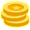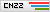# 分析DEF CON CTF Quals 2020中Crypto方向题目

|## 前言

DEF CON CTF Quals 2020中共有三道CRYPTO方向题目（两道CRYPTO、一道RE+CRYPTO），题目难度适中，比赛期间三道题目都出现了非预期解，本文对这三道题目的预期解和非预期解都进行一下分析。

## coooppersmith

### 题目描述

I was told by a cooopersmith that I should send hackers encrypted messages because it is secure.
coooppersmith.challenges.ooo 5000

130/500 pts, 41 solves

### 解题思路

``````Please input prefix IN HEX with length no more than 120: deadbeef
-----BEGIN RSA PUBLIC KEY-----
MIIBCQKCAQA72hJsgSuPfUh1+EVHJMilCiEw5W5IiWOjdfRnuqdn2rsuvJSazeUg
3X0MITlZbIgM9oh7KP3uk6cGjF1HyOI1eAE4Yf8zLXHfVbFg5cx5ZcIJ7twrFgIV
Bz8kwTim8nt1bNf3AwPXpMJFr23BLMV6qaAGFcoffxgcEVz6hrHZDJYDXRfDlLGN
8a2x2w45dTLy53HR5GKV4xE4JoY9bJp7zmdJ/AVPD241XcHxDCaYh6qKeOjPAXLM
MJXR4qjHNfOJ9clzn57+V0553dPOkwsTdhtnjLo1lCuNurHinfiqQgpvJF4p7T2R
n3YE9HhPghwbiX6wwunBP4x5qI0qn47hAgMBAAE=
-----END RSA PUBLIC KEY-----
Question:
``````

`sub_1E25`函数部分代码：

`sub_18C4`函数部分代码：

`sub_18C4`函数的返回值为`r`，用户输入的16进制串的10进制整数形式为`u`，用户输入的16进制串的长度为`l``x`是区间`[ 0, 2^(2 * (128 - l)) )`上的一个随机数，则有：

`sub_14D8`函数部分代码：

`sub_165E`函数部分代码：

`sub_1B3E`函数部分代码：

`f(x) = 2 * r * x + 1, x in Zmod(N)`，然后我们仍然可以穷举`r`，此时我们可以通过Coppersmith相关攻击中计算计小整数解的方式来获取到`x`的值（也即`k_0`的值，`k_1`同理），POC如下（注意在SageMath下使用`small_roots`方法计算小整数解时要求多项式为首一多项式，因此我们需要将多项式形式做一个变形）：

``````sage: P.<x> = PolynomialRing(Zmod(N))
....: f = x + inverse_mod(2 * r, N)
....: res = f.small_roots(X = r, beta = 0.4)
....: k_0 in res
....:
True
``````

p.s.

### 解题脚本

``````#!/usr/bin/env sage

import rsa
from gmpy2 import iroot
from pwn import *
from Crypto.Util.number import *

io = remote('coooppersmith.challenges.ooo', 5000)
#io = process("./service")

user_input = '000000000000000000000000000000000000000000000000000000000000111111111111111111111111111111111111111111111111111111111111'

io.sendline(user_input)
_ = io.recvline()
pkeydata = io.recvuntil("-----END RSA PUBLIC KEY-----\n")
_ = io.recvline()
sum_ct = int(io.recvline().strip(), 16)

N = pubkey.n
e = pubkey.e

for x in range(0, 2**16):
r = int(user_input, 16) * 2**32 + x
if (N - 1) % r == 0:
break

P.<x> = PolynomialRing(Zmod(N))
f = x + inverse_mod(2 * r, N)
res = f.small_roots(X = r, beta = 0.4)
k_0 = int(res)
p = 2 * r * k_0 + 1
q = N // p

d = inverse(e, (p - 1) * (q - 1))
sum_pt = pow(sum_ct, d, N)

tmp = long_to_bytes(sum_pt)
tmp = tmp[:-1].split(b"of ").split(b" and ")
sum = int(tmp) + int(tmp)

io.sendline(str(sum))
_ = io.recvline()
ct = int(io.recvline().strip(), 16)
pt = pow(ct, d, N)

FLAG = long_to_bytes(pt)
print(FLAG)

# OOO{Be_A_Flexible_Coppersmith}
``````

## notbefoooled

### 题目描述

What’s the trick to not be foooled?
notbefoooled.challenges.ooo 5000
143/500 pts, 30 solves

### 解题思路

p.s.

``````Eqp = EllipticCurve(Qp(p, 8), [ZZ(t) for t in E.a_invariants()])
``````

``````Eqp = EllipticCurve(Qp(p, 2), [ ZZ(t) + randint(0,p)*p for t in E.a_invariants() ])
``````

### 解题脚本

``````#!/usr/bin/env sage

from pwn import *

'''
BITS = 113

def p_gen(BITS):
for i in range(2**BITS, 2**(BITS + 1)):
koeff = 1 + 3 * i * i
if koeff % 4 != 0:
continue
p = koeff // 4
if not is_prime(p):
continue
j_invar = 0
a = 0
b = (54 * 3 * pow(3 * 12**3, inverse_mod(2, p), p)) % p
E = EllipticCurve(GF(p), [a, b])
if p == E.order():
return (a, b, p)
'''

a = 0
b = 839808
p = 80879840001451919384001045261062739512883607574894458541090782884541
E = EllipticCurve(GF(p), [a, b])

io = remote("notbefoooled.challenges.ooo", 5000)

io.sendlineafter("a = ", str(a))
io.sendlineafter("b = ", str(b))
io.sendlineafter("p = ", str(p))

_ = io.recvuntil(b"generator: (")
tmp = io.recvline().strip().replace(b')', b'').split(b", ")
G = E(int(tmp), int(tmp))

k = randrange(1, p)
P = k * G

io.sendlineafter("x = ", str(P))
io.sendlineafter("y = ", str(P))

io.recv()

# OOO{be_Smarter_like_you_just_did}
``````

## ooo-flag-sharing

### 题目描述

Share flags safely with the latest OOO service!
ooo-flag-sharing.challenges.ooo 5000

135/500 pts, 36 solves

### 解题思路

• 功能1

• 功能2

• 功能3

• 功能4

``````01111011010011110100111101001111
``````

### 解题脚本

``````#!/usr/bin/env python3

from pwn import *
from math import gcd
from Crypto.Util.number import *

def fun_1(io, secret, num):
io.sendlineafter('Choice: ', '1')
io.sendlineafter('Enter secret to share: ', secret)
_ = io.recvuntil("Your secret's ID is: ")
secret_id = io.recvline().strip()
io.sendlineafter('Number of shares to make: ', str(num))
_ = io.recvuntil('Your shares are: ')
shares = eval(io.recvline().strip())
return secret_id, shares

def fun_2(io, secret_id, shares):
io.sendlineafter('Choice: ', '2')
io.sendlineafter("Enter the secret's ID: ", secret_id)
io.sendlineafter('Enter your shares of the secret: ', str(shares))
_ = io.recvuntil('Your secret is: ')
secret = io.recvline().strip()
secret = eval(secret)
return secret

def fun_3(io):
io.sendlineafter('Choice: ', '3')
io.recvuntil("Our secret's ID is: ")
secret_id = io.recvline().strip()
shares = eval(io.recvline().strip())
return secret_id, shares

def fun_4(io, secret_id, shares):
io.sendlineafter('Choice: ', '4')
io.sendlineafter("Enter the secret's ID: ", secret_id)
io.sendlineafter("Enter your shares of the secret: ", str(shares))
res = io.recvline()
return res.startswith(b"Congrats!")

def recover_P(io):
input_a = b'~' * 40
input_b = b'~' * 41
secret_id, shares = fun_1(io, input_a, 5)
secret_a = fun_2(io, secret_id, shares)
secret_id, shares = fun_1(io, input_b, 5)
secret_b = fun_2(io, secret_id, shares)
kp = int.from_bytes(input_a, 'big') - int.from_bytes(secret_a, 'little')
gp = int.from_bytes(input_b, 'big') - int.from_bytes(secret_b, 'little')
P = gcd(kp, gp)
if not isPrime(P):
for i in range(2, 100, 2):
if isPrime(P // i):
P = P // i
break
return P

def recover_m_e(io, secret_id, shares, P):
known_idx = [share for share in shares]
for a in range(1, 100):
for b in range(a + 1, 100):
if (a in known_idx) or (b in known_idx):
continue
m_e = int.from_bytes(fun_2(io, secret_id, [(a, 0), (b, 0), (known_idx, 0), (known_idx, 0), (known_idx, 1)]), 'little')
token = fun_4(io, secret_id, shares[:-1] + [(shares, (shares + (1 << 32) * inverse(m_e, P)) % P)])
if token:
return m_e

def binary_search_x(io, secret_id, shares, P, m_e):
low = 0
high = P >> 32
while low <= high:
x = (low + high) // 2
token = fun_4(io, secret_id, shares[:-1] + [(shares, (shares + (x << 32) * inverse(m_e, P)) % P)])
if token:
low = x + 1
else:
high = x - 1
return x

if __name__ == "__main__":
io = remote("ooo-flag-sharing.challenges.ooo", 5000)
secret_id, shares = fun_3(io)
P = recover_P(io)
m_e = recover_m_e(io, secret_id, shares, P)
x = binary_search_x(io, secret_id, shares, P, m_e)
FLAG = b"OOO{" + (P - (x << 32)).to_bytes(32, 'little')[4:]
print(FLAG)

# OOO{ooo_c4nt_ke3p_secr3ts!}
``````

## 参考资料

0赞道路结冰

## 发表评论### TA的文章

• ##### 分析N1CTF 2021中Crypto方向题目

2021-12-06 14:30:36

• ##### 分析DEF CON CTF Quals 2020中Crypto方向题目

2021-03-02 15:30:18

• ##### 分析ASIS CTF 2020中Crypto方向题目

2020-10-20 16:30:59

• ##### 七夕-分析zer0pts CTF 2020中Crypto方向题目

2020-08-27 10:30:13

• ##### 分析BSidesSF CTF 2020中Crypto方向题目

2020-03-18 11:00:01

### 相关文章

• ##### 培养云上安全人才 | 阿里云2023首届CTF大赛重磅启动

2023-04-24 19:17:46

• ##### Ichunqiu云境 —— Exchange Writeup

2023-03-03 14:30:36

• ##### Ichunqiu云境 - Delegation Writeup

2023-01-06 10:30:50

• ##### Ichunqiu云境 —— Tsclient Writeup

2023-01-05 10:30:31

• ##### 活动 | 长亭科技2023第五届 Real World CTF 战火已燃，等你来战！

2022-12-21 17:00:34

• ##### 从一道题入门 UEFI PWN

2022-11-11 15:30:05

• ##### 2022年工业信息安全技能大赛“望岳杯”锦标赛 wp

2022-10-31 15:30:36

### 热门推荐

• 投稿须知
• 转载须知
• 官网QQ群8：819797106
• 官网QQ群3：830462644(已满)
• 官网QQ群2：814450983(已满)
• 官网QQ群1：702511263(已满)
Copyright © 北京奇虎科技有限公司 360网络攻防实验室 安全客 All Rights Reserved 京ICP备08010314号-66